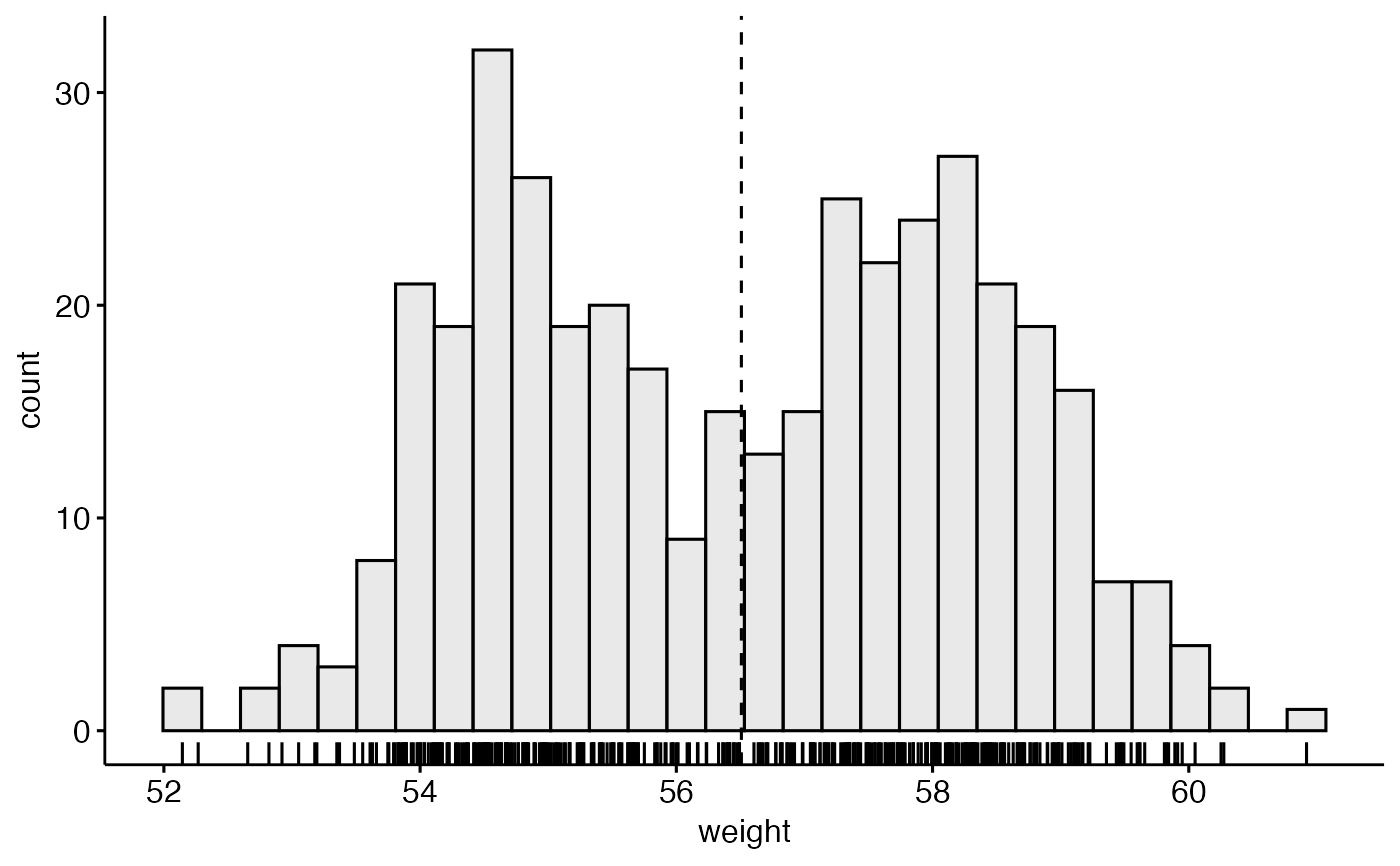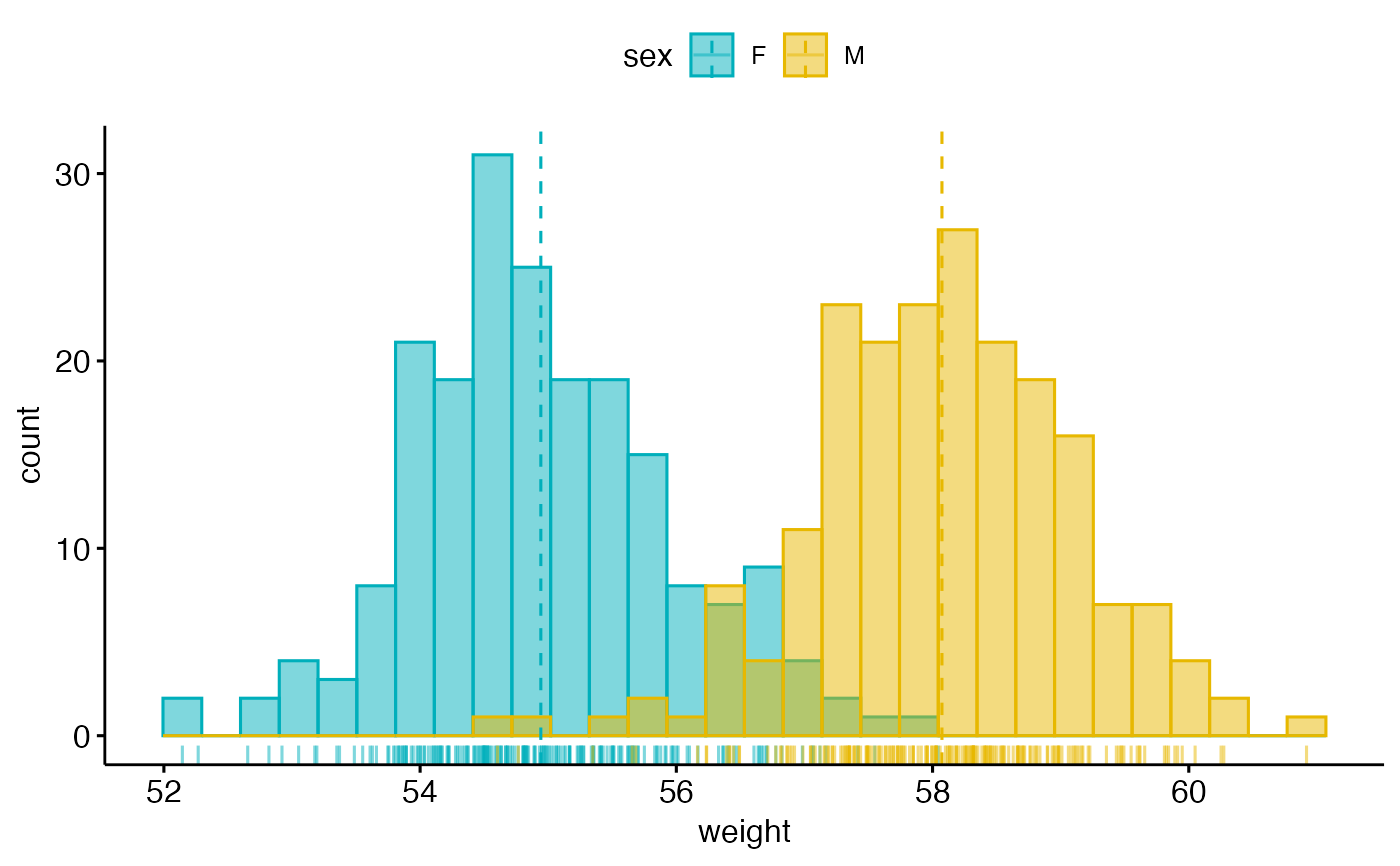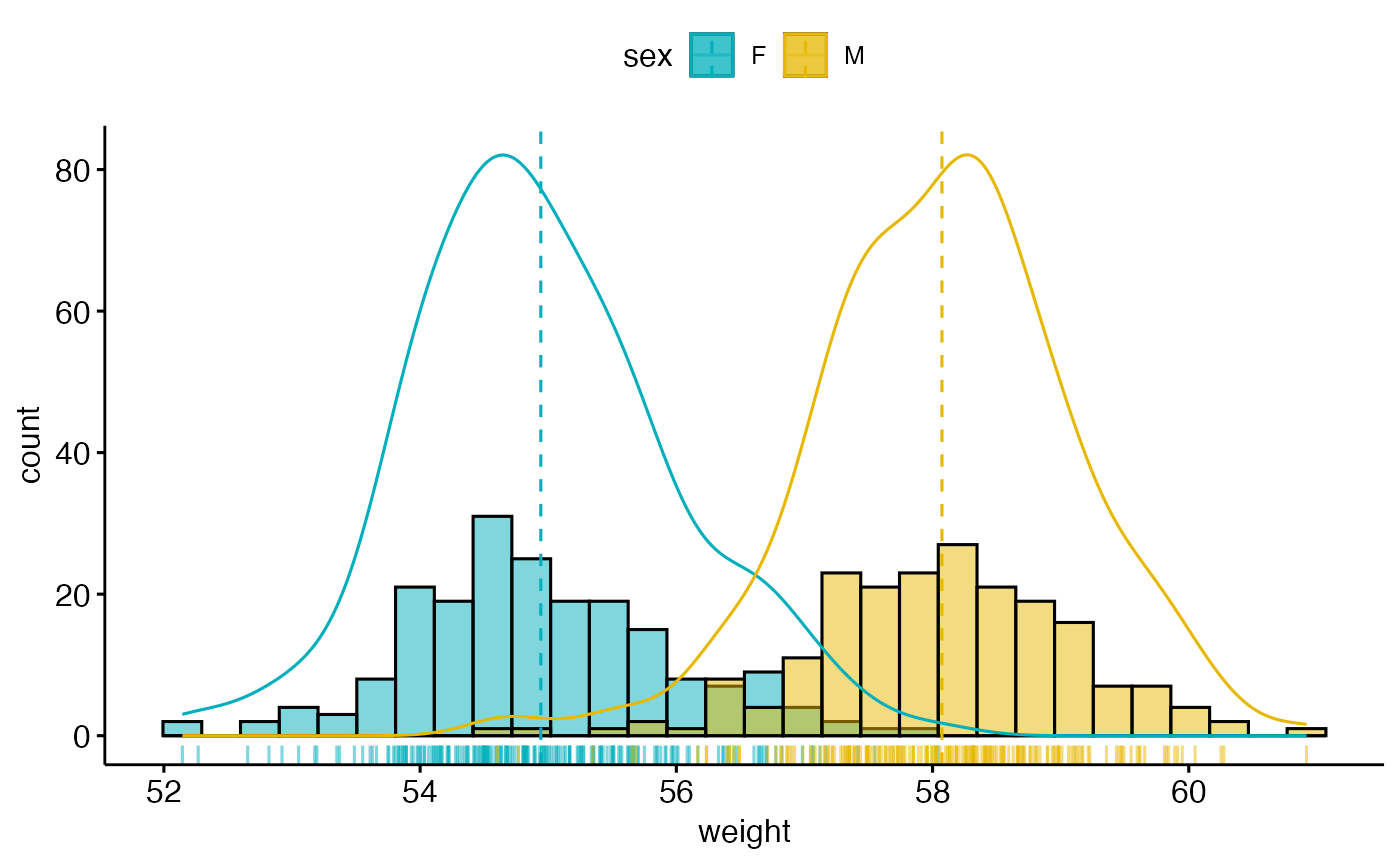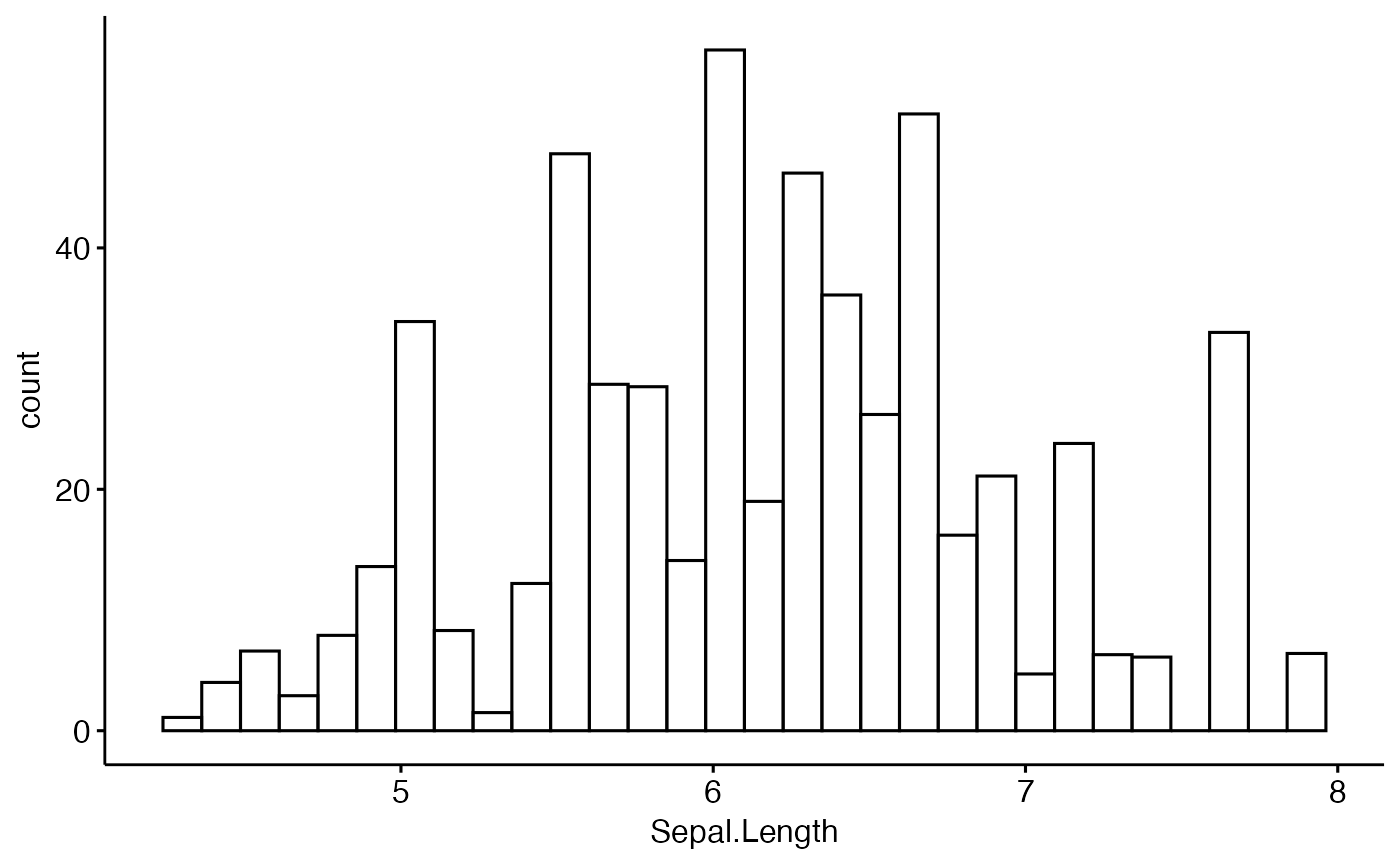Create a histogram plot.

gghistogram(
data,
x,
y = "count",
combine = FALSE,
merge = FALSE,
weight = NULL,
color = "black",
fill = NA,
palette = NULL,
size = NULL,
linetype = "solid",
alpha = 0.5,
bins = NULL,
binwidth = NULL,
title = NULL,
xlab = NULL,
ylab = NULL,
facet.by = NULL,
panel.labs = NULL,
short.panel.labs = TRUE,
rug = FALSE,
label = NULL,
font.label = list(size = 11, color = "black"),
label.select = NULL,
repel = FALSE,
label.rectangle = FALSE,
position = position_identity(),
ggtheme = theme_pubr(),
...
)

## Arguments

data

a data frame

x

variable to be drawn.

y

one of "density" or "count".

combine

logical value. Default is FALSE. Used only when y is a vector containing multiple variables to plot. If TRUE, create a multi-panel plot by combining the plot of y variables.

merge

logical or character value. Default is FALSE. Used only when y is a vector containing multiple variables to plot. If TRUE, merge multiple y variables in the same plotting area. Allowed values include also "asis" (TRUE) and "flip". If merge = "flip", then y variables are used as x tick labels and the x variable is used as grouping variable.

weight

a variable name available in the input data for creating a weighted histogram.

color, fill

histogram line color and fill color.

palette

the color palette to be used for coloring or filling by groups. Allowed values include "grey" for grey color palettes; brewer palettes e.g. "RdBu", "Blues", ...; or custom color palette e.g. c("blue", "red"); and scientific journal palettes from ggsci R package, e.g.: "npg", "aaas", "lancet", "jco", "ucscgb", "uchicago", "simpsons" and "rickandmorty".

size

Numeric value (e.g.: size = 1). change the size of points and outlines.

linetype

line type. See show_line_types.

alpha

numeric value specifying fill color transparency. Value should be in [0, 1], where 0 is full transparency and 1 is no transparency.

bins

Number of bins. Defaults to 30.

binwidth

numeric value specifying bin width. use value between 0 and 1 when you have a strong dense dotplot. For example binwidth = 0.2.

title

plot main title.

xlab

character vector specifying x axis labels. Use xlab = FALSE to hide xlab.

ylab

character vector specifying y axis labels. Use ylab = FALSE to hide ylab.

facet.by

character vector, of length 1 or 2, specifying grouping variables for faceting the plot into multiple panels. Should be in the data.

panel.labs

a list of one or two character vectors to modify facet panel labels. For example, panel.labs = list(sex = c("Male", "Female")) specifies the labels for the "sex" variable. For two grouping variables, you can use for example panel.labs = list(sex = c("Male", "Female"), rx = c("Obs", "Lev", "Lev2") ).

short.panel.labs

logical value. Default is TRUE. If TRUE, create short labels for panels by omitting variable names; in other words panels will be labelled only by variable grouping levels.

allowed values are one of "mean" or "median" (for adding mean or median line, respectively).

parameters (color, size, linetype) for the argument 'add'; e.g.: add.params = list(color = "red").

rug

logical value. If TRUE, add marginal rug.

logical value. If TRUE, add density curves.

label

the name of the column containing point labels. Can be also a character vector with length = nrow(data).

font.label

a list which can contain the combination of the following elements: the size (e.g.: 14), the style (e.g.: "plain", "bold", "italic", "bold.italic") and the color (e.g.: "red") of labels. For example font.label = list(size = 14, face = "bold", color ="red"). To specify only the size and the style, use font.label = list(size = 14, face = "plain").

label.select

can be of two formats:

• a character vector specifying some labels to show.

• a list containing one or the combination of the following components:

• top.up and top.down: to display the labels of the top up/down points. For example, label.select = list(top.up = 10, top.down = 4).

• criteria: to filter, for example, by x and y variabes values, use this: label.select = list(criteria = "y > 2 & y < 5 & x %in% c('A', 'B')").

repel

a logical value, whether to use ggrepel to avoid overplotting text labels or not.

label.rectangle

logical value. If TRUE, add rectangle underneath the text, making it easier to read.

position

Position adjustment, either as a string, or the result of a call to a position adjustment function. Allowed values include "identity", "stack", "dodge".

ggtheme

function, ggplot2 theme name. Default value is theme_pubr(). Allowed values include ggplot2 official themes: theme_gray(), theme_bw(), theme_minimal(), theme_classic(), theme_void(), ....

...

other arguments to be passed to geom_histogram and ggpar.

## Details

The plot can be easily customized using the function ggpar(). Read ?ggpar for changing:

• main title and axis labels: main, xlab, ylab

• axis limits: xlim, ylim (e.g.: ylim = c(0, 30))

• axis scales: xscale, yscale (e.g.: yscale = "log2")

• color palettes: palette = "Dark2" or palette = c("gray", "blue", "red")

• legend title, labels and position: legend = "right"

• plot orientation : orientation = c("vertical", "horizontal", "reverse")

ggdensity and ggpar

## Examples

# Create some data format
set.seed(1234)
wdata = data.frame(
sex = factor(rep(c("F", "M"), each=200)),
weight = c(rnorm(200, 55), rnorm(200, 58)))

#>   sex   weight
#> 1   F 53.79293
#> 2   F 55.27743
#> 3   F 56.08444
#> 4   F 52.65430

# Basic density plot
# Add mean line and marginal rug
gghistogram(wdata, x = "weight", fill = "lightgray",
add = "mean", rug = TRUE)
#> Warning: Using bins = 30 by default. Pick better value with the argument bins.
#> Warning: geom_vline(): Ignoring mapping because xintercept was provided.
#> Warning: geom_vline(): Ignoring data because xintercept was provided.# Change outline colors by groups ("sex")
# Use custom color palette
gghistogram(wdata, x = "weight",
add = "mean", rug = TRUE,
color = "sex", palette = c("#00AFBB", "#E7B800"))
#> Warning: Using bins = 30 by default. Pick better value with the argument bins.# Change outline and fill colors by groups ("sex")
# Use custom color palette
gghistogram(wdata, x = "weight",
add = "mean", rug = TRUE,
color = "sex", fill = "sex",
palette = c("#00AFBB", "#E7B800"))
#> Warning: Using bins = 30 by default. Pick better value with the argument bins.# Combine histogram and density plots
gghistogram(wdata, x = "weight",
add = "mean", rug = TRUE,
fill = "sex", palette = c("#00AFBB", "#E7B800"),
#> Warning: Using bins = 30 by default. Pick better value with the argument bins.#> Warning: Using bins = 30 by default. Pick better value with the argument bins.